### Gas Pipe (Fanno)

The gas pipe element of type Fanno is a pipe element with constant cross section (Figure 90), for which the Fanno formulae are applied .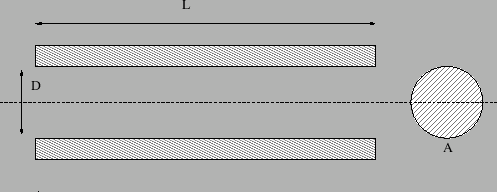The friction parameter is determined as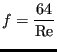(61)

for laminar flow ( Re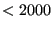) and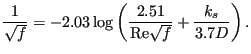(62)

for turbulent flow. Here,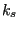is the diameter of the material grains at the surface of the pipe and Re is the Reynolds number defined by

 Re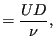(63)

where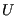is the fluid velocity and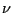is the kinematic viscosity.

It is described by the following parameters (to be specified in that order on the line beneath the *FLUID SECTION,TYPE=GAS PIPE FANNO ADIABATIC or *FLUID SECTION,TYPE=GAS PIPE FANNO ISOTHERMAL card):

• A: cross section of the pipe element
• D: diameter of the pipe element
• L: length of the pipe element
•: grain diameter at the pipe surface
• form factor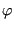• oil mass flow in the pipe (only if the OIL parameter is used to define the kind of oil in the *FLUID SECTION card)
• not used (internally: oil material number)

The default gas pipe is adiabatic, i.e. there is no heat exchange with the pipe. Alternatively, the user may specify that the pipe element is isothermal. This means that the static temperature does not change within the pipe. In that case the energy equation in one of the two end nodes of the element is replaced by an isothermal condition.

The form factoris only used to modify the friction expression for non-circular cross sections in the laminar regime as follows: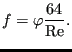(64)

Values forfor several cross sections can be found in . For a square cross section its value is 0.88, for a rectangle with a height to width ratio of 2 its value is 0.97.

Instead of specifying a fixed diameter and length, these measures may also be calculated from the actual position of given nodes. The version in which the radius is calculated from the actual distance between two nodes a and b is described by the following parameters (to be specified in that order on the line beneath the *FLUID SECTION,TYPE=GAS PIPE FANNO ADIABATIC FLEXIBLE RADIUS or *FLUID SECTION,TYPE=GAS PIPE FANNO ISOTHERMAL FLEXIBLE RADIUS card):

• node number a
• node number b
• L: length of the pipe
•: grain diameter at the pipe surface
• form factor• oil mass flow in the pipe (only if the OIL parameter is used to define the kind of oil in the *FLUID SECTION card)
• not used (internally: oil material number)

The version in which the radius is calculated from the actual distance between two nodes a and b and the length from the actual distance between nodes a and c is described by the following parameters (to be specified in that order on the line beneath the *FLUID SECTION,TYPE=GAS PIPE FANNO ADIABATIC FLEXIBLE RADIUS AND LENGTH or *FLUID SECTION,TYPE=GAS PIPE FANNO ISOTHERMAL FLEXIBLE RADIUS AND LENGTH card):

• node number a
• node number b
• node number c
•: grain diameter at the pipe surface
• form factor• oil mass flow in the pipe (only if the OIL parameter is used to define the kind of oil in the *FLUID SECTION card)
• not used (internally: oil material number)

Although a gas pipe looks simple, the equations for compressible flow in a pipe are quite complicated. Here, the derivation is first presented for the adiabatic case. Starting from the energy equation (38) and using the relation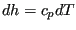for an ideal gas one arrives at: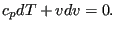(65)

By means of the definition of the Mach number (32) one gets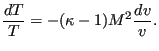(66)

Because of the ideal gas equation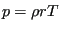this can also be written as: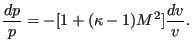(67)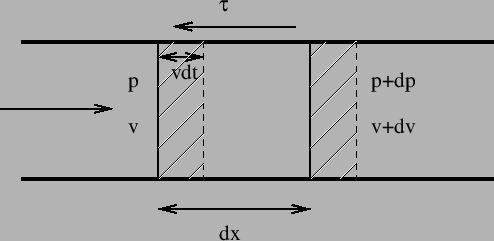Looking at Figure (91) the momentum equation can be derived by applying Newton's second law, which states that the sum of the forces is the change of momentum (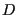is the diameter of the pipe,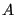its cross section):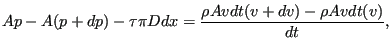(68)

or, using Darcy's law (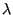is the Darcy friction factor)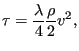(69)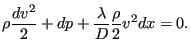(70)

One can remove the density by means of the gas equation arriving at: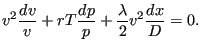(71)

Combining this with what was obtained through the energy equation (67) leads to (removing p in this process):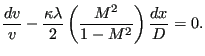(72)

This equation contains both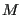and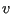. We would like to get an equation with only one of these parameters. To this end the equation defining the Mach number (32) is differentiated and the energy equation in the form (65) is used to remove T, leading to: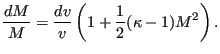(73)

In that way, the previous equation can be modified its final form: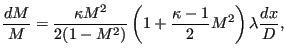(74)

expressing the Mach number as a function of the distance along the pipe. This equation tells us that for subcritical flow (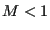) the Mach number increases along the pipe whereas for supercritical flow (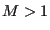) the Mach number decreases. Consequently, the flows tends to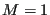along the pipe. Notice that by assigning the sign oftothe above equation also applies to negative velocities. Substituting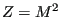and integrating both sides yields: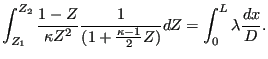(75)

Since (partial fractions)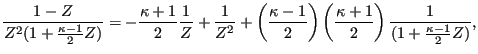(76)

one obtains finally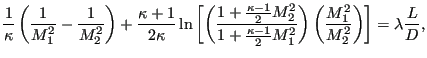(77)

linking the Mach number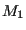at the start of the pipe with the Mach number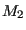at the end of the pipe, the pipe length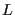and the Darcy friction coefficient.

Notice that Equation (71) can be used to calculate an estimate of the mass flow due to a given pressure gradient by assuming incompressibility. For an incompressibile medium in a pipe with constant cross section the velocity is constant too (mass conservation) and Equation (71) reduces to: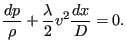(78)

Integrating yields: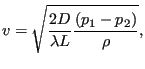(79)

or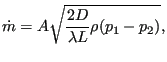(80)

which can finally also be written as: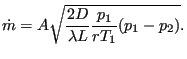(81)

For an estimate of the mass flow in the gas pipe the above static variables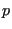and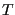are replaced by the total variables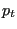and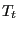, respectively. Equation (77) is the governing equation for an adiabatic gas pipe. In order to apply the Newton-Raphson procedure (linearization of the equation) with respect to the variables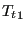,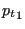,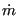,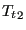and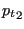, this equation is first derived w.r.tand(denoting the equation by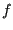; the derivation is slightly tedious but straightforward):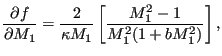(82)

and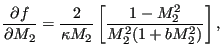(83)

where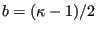. Now,at position 1 and 2 is linked to,andat the same location through the general gas equation: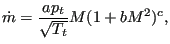(84)

where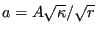and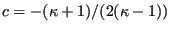. Careful differentiation of this equation leads to the surprisingly simple expression: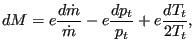(85)

where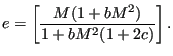(86)

Finally, the chain rule leads to the expressions looked for: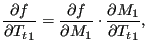(87)

etc.

For the isothermal pipe the ideal gas equation leads to: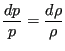(88)

and from the mass conservation, Equation (43) one gets: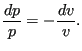(89)

Furthermore, the definition of the Mach number yields: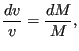(90)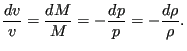(91)

By substituting these relationships and the definition of the Mach number one can reduce all variables in Equation (71) by the Mach number, leading to: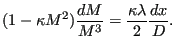(92)

This equation shows that for an isothermal gas pipe the flow tends to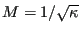and not to 1 as for the adiabatic pipe. Substitutingand integrating finally yields: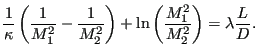(93)

The above equation constitutes the element equation of the isothermal gaspipe. Applying the Newton-Raphson procedure requires the knowledge of the derivatives w.r.t. the basis variables. The procedure is similar as for the adiabatic gas pipe. The derivative of the element equaton w.r.t.andis easily obtained (denoting the left side of the above equation by):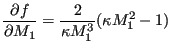(94)

and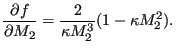(95)

The use of an isothermal gas pipe element, however, also requires the change of the energy equation. Indeed, in order for the gas pipe to be isothermal heat has to be added or subtracted in one of the end nodes of the element. The calculation of this heat contribution is avoided by replacing the energy equation in the topologically downstream node (or, if this node has a temperature boundary condition, the topologically upstream node) by the requirement that the static temperature in both end nodes of the element has to be the same. This is again a nonlinear equation in the basic unknowns (total temperature and total pressure in the end nodes, mass flow in the middle node) and has to be linearized. In order to find the derivatives one starts from the relationship between static and total temperature: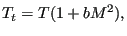(96)

where. Differentiation yields: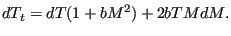(97)

Replacing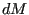by Equation (85) finally yields an expression in which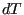is expressed as a function of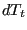,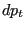and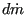.

Example files: gaspipe10, gaspipe8-cfd-massflow, gaspipe8-oil.